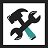Complete Tools

# CAGR Calculator

Calculate Compound Annual Growth Rate and its components

Categories
Finance Tools
Calculate
Initial Value
Final Value
Duration
CARG (Growth Rate) %

## How Does CAGR Calculator Work?

The Compound Annual Growth Rate (CAGR) calculator tool allows you to compute CAGR and its components such as growth rate, duration, and initial or end value. This is helpful whether you're thinking about expanding your business or investing.

Any component of compound growth can be computed rapidly, and the growth can be seen as a chart. This compound interest growth chart is also available as a PNG image, which you can use to share with others or keep as a reference.

The following is a list of the many components of compounded annual growth, along with formulas for calculating them:

• Initial Value = The amount invested at the start, commonly known as the principal.
• Duration = The amount of time (in years) that the money is invested for, also known as a period.
• CAGR = The annual growth rate (in percent) of interest added.
• Final Value = The total amount after factoring in annual growth. This is also referred to as the accrued amount or the (principal + interest) amount.

## CAGR formula

The compound annual growth rate (CAGR) is determined for an initial value that has been subjected to compound interest for a set period of time. You may determine the growth rate (CAGR) using the CAGR formula below if you know the initial amount, final amount, and investment length.

CAGR = ((Final Value / Initial Value)(1 / Duration) - 1) * 100

## Initial Value formula

When you know the ultimate amount, the duration of the investment, and the CAGR, you can compute the Initial Value in compound interest over a defined period. The initial value can be calculated using the formula below.

Initial Value = Final Value / (1 + (CAGR / 100))Duration

## Final Value formula

For a given initial amount, CAGR, and term, the Final Value is calculated in compound interest. This is also known as a compound interest calculation or a reverse CAGR calculation of an investment's accrued amount. The formula below can be used to calculate the final value.

Final Value = Initial Value * (1 + (CAGR / 100))Duration

## Duration formula

When you know the initial amount, the end amount, and the CAGR, you can compute the duration in compound interest. Using the method below, you can determine how long the growth will last.

Duration = ln(Final Value / Initial Value) / ln(1 + CAGR/100)

Here `ln` denotes natural logarithm, or loge.

## How to Use CAGR Calculator?

• You can calculate CAGR by entering the starting value, ending value, and compound annual growth period.
• The CAGR calculator will calculate and display the percent value of the CAGR (growth rate) in real time.
• To compute any other component, click on the label that corresponds to it, such as Initial Value, Final Value, or Duration.
• While you can enter alternative values, the result that is presently being calculated will be highlighted and become non-editable.
• For the calculations, please refer to the formula described in the next section.

## Credits

• Recharts: Redefined chart library built with React and D3.